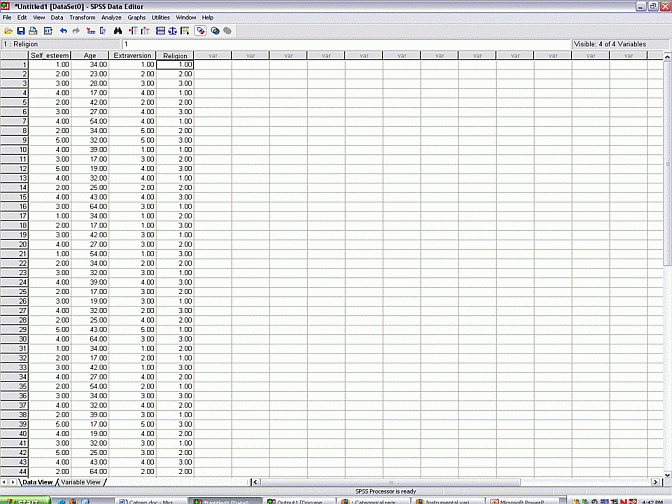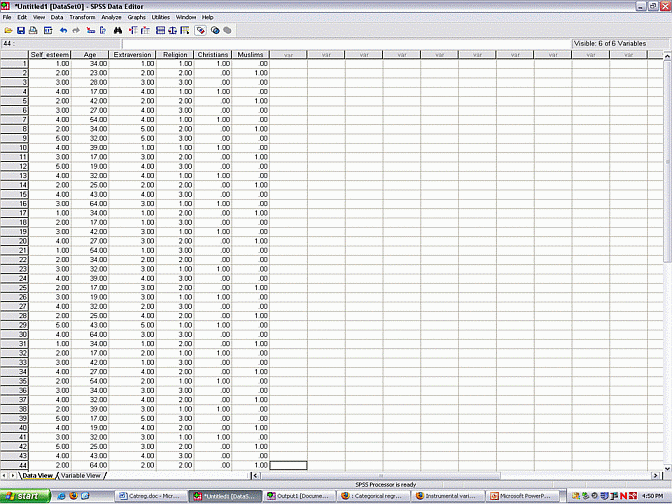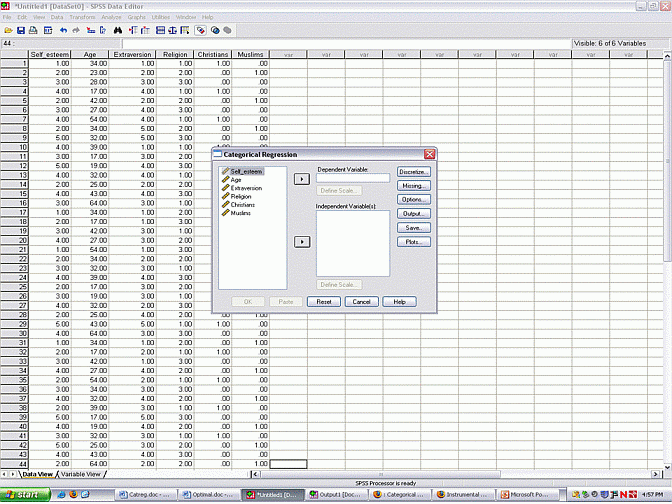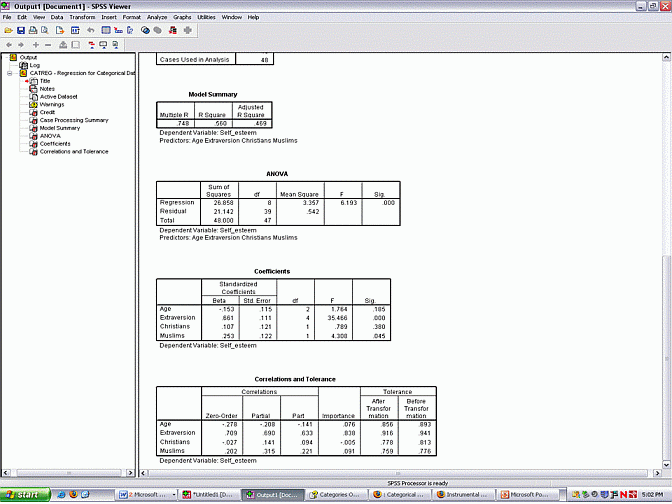# Categorical regression analysis

### Introduction

Categorical regression mirrors conventional multiple regression, except this technique can also accommodate nominal and ordinal variables. In particular, nominal and ordinal variables are effectively transformed into interval variables. Multiple regression analysis is then applied to these transformed variables.

#### Ordinal variables in traditional regression

To illustrate categorical regression, suppose a researcher determines the self esteem, age, extroversion, and religion in a sample of individuals. An extract of the data are displayed below.The researcher wants to ascertain whether or not self esteem is influenced by age, extroversion, or religion. To fulfill this objective, the researcher would prefer to undertake a multiple regression. Unfortunately, two of the variables are ordinal and one of the variables is nominal. Multiple regression, therefore, is sometimes considered unsuitable under these circumstances.

To illustrate this problem, first consider the ordinal variables. Multiple regression will assume that consecutive levels of these variables are equal. For instance, the difference between 1 and 2 on self esteem is regarded as equivalent to the difference between 3 and 4.

These numbers, however, are arbitrary. For instance, the researcher could have justifiably utilized the numbers 3, 7, 100, 430, 1094 instead of 1, 2, 3, 4, and 5. Unfortunately, each scale will generate an entirely different pattern of results. The question, then, is which scale yields the correct solution: the scale that entails 3, 7, 100, 4430, 1094 , the scale that entails 1, 2, 3, 4, 5, or another one of the endless number of scales that could have been applied?

#### Nominal variables in traditional regression

As discussed in the previous section, the scale of ordinal variables is arbitrary, which presents an interesting problem to multiple regression analysis. Nominal variables also pose a problem to multiple regression. For instance, consider the variable labeled as religion. Suppose that 's represent Christians, '2's represent Muslims, and so forth.

This variable, unless modified appropriately, obviously cannot be entered into the multiple regression. Otherwise, the procedure will assume that religion is quantitative, and thus regard Muslims as higher on some trait than Christians--a meaningless assumption.

Fortunately, nominal variables can be entered in traditional regression, provided they are transformed appropriately. In particular, the researcher needs to dummy code the variable. In essence, this process involves creating a separate column for each religion, apart from one religion, which will be denoted as the reference category. The upshot of this process is depicted below For example, in the column labeled Christians, 1s denote Christians and 0s denote non-Christians. Likewise, in the column labeled Muslims, 1s denote Muslims and 0s denote non-Muslims. Participants coded as 0 on all the religions must pertain to the reference category - that is, the religion that was not coded, such as Buddhists.When these dummy variables are entered into traditional regression, the output will provide information about the relationship between religion and self esteem. For instance, suppose the variable labeled as 'Christians' attains significance. Roughly speaking, this finding would indicate that Christians differ from Buddhists, the reference category, on self-esteem. Likewise, suppose the variable labeled as 'Muslims' does not attain significance. This finding would indicate that Muslims do not differ from Buddhists on self-esteem.

This discussion neglects some of the complexities associated with dummy coding, but is sufficient to demonstrate one of the shortfalls of this approach. For example, not all of the religions have been compared. For instance, the output does not reveal whether or not Christians differ significantly from Muslims. To undertake this comparison, a different coding scheme would have to be undertaken. However, this coding scheme may neglect some other vital comparisons. Traditional regression does not permit the researcher to undertake all possible comparisons.

### Categorical regression analysis

#### The rationale

To reiterate, ordinal and nominal variables can undermine traditional regression. For ordinal variables, the scale is arbitrary and yet different scales yield disparate findings. For nominal variables, the output is difficult to interpret and may not provide information about all of the relevant comparisons.

Fortunately, categorical regression analysis, one of the options in SPSS, circumvents these problems. Essentially, categorical regression converts nominal and ordinal variables to interval scales. This conversion is designed to maximize the relationship between each predictor and the dependent variable. To appreciate this transformation, see Overview of Optimal Scaling, which is an article that is currently being constructed.

### Implementing categorical regression analysis with SPSS

To implement this technique,

• Select the "Analyze" menu, the "Regression" option, and finally "Optimal Scaling". The following dialogue box emerges• Specify the dependent and independent variables in the appropriate boxes.
• You now have to specify the scale and range of each variable. To this end, highlight the variable and then press "Define scale" to open the dialogue box.
• In this dialogue box, specify the level of measurement associated with this variable: nominal, ordinal, or numerical. Numerical is synonymous with interval or ratio. You can use the "spline" alternatives if you prefer a smoother, but less accurate fit.
• Finally, press 'OK' to execute the program.

The only complication relates to specifying the level of measurement. In general, ordinal variables are specified as ordinal, nominal variables are specified as nominal, and so forth Nonetheless, exceptions to this principle exist.

For instance, consider two interval variables that are related in a non-linear fashion. To optimize the relationship between these variables, the researcher may designate one of them as nominal or ordinal. As a consequence, SPSS will modify the scale of this variable to optimize the relationship.

In other words, when the researcher does not want to modify the spacing between consecutive levels, the variable should be designated as "Numeric". When the researcher wants to modify the spacing between consecutive levels, without adjusting the order, the variable should be designated as "Ordinal". Otherwise, the variable should be designated as "Nominal".

#### Interpreting the output

The output generated by categorical regression is similar to the output generated by traditional regression, although certain complications need to be recognized. The final table, presented below, contains the most crucial information.Traditional regression provides the unstandardized beta values, standard error, standardized beta values, t value and p value associated with each predictor. Categorical regression differs in the following respects:

• No unstandardized beta values are provided, because all variables are standardized before the process is undertaken
• F values are provided instead of t values. Actually, F simply equals t squared. This difference is thus trivial.
• Additional indices are also provided, including partial and part correlations. These indices are described below.

The zero-order correlation is simply the correlation between each predictor and the dependent variable, after these variables have undergone the appropriate transformations.

Importance obviously indicates the importance of each predictor, using Pratt's measure. This measure is roughly equivalent to the product of the regression coefficient and zero-order correlation. This index is primarily used to uncover suppressor variables. That is, suppose a predictor yields a relatively high beta but low importance. This situation suggests the variable may have been suppressed by other predictors.

A clear exposition of partial correlations, part correlations, and tolerance can be located in most multivariate textbooks. In essence, partial and part correlations are like zero-order correlations, except the effect of all other predictors has been controlled. Tolerance is utilized to identify multicollinearity.

To generate some additional tables or execute more complex procedures, press the "Options" button, and tick the appropriate box.

• You can choose between "Numerical" and "Random" configurations. The 'Random' configuration should be selected whenever any of the variables are nominal.
• By default, SPSS will exclude all participants that generated one or more missing values. You can choose "Mode imputation" instead, which substitutes the mode of that variable for each missing value. When none of these options are appropriate, you can use the program "Missing value analysis" to execute more appropriate substitutions.
• By selecting the "Quantifications" box, SPSS will present information about the transformations. For instance, SPSS may reveal that a scale initially coded as 1, 2, 3, 4, and 5 was transformed to an interval scale coded as 1, 4, 9, 12, 40.
• You can also save these transformed scales in the data sheet.